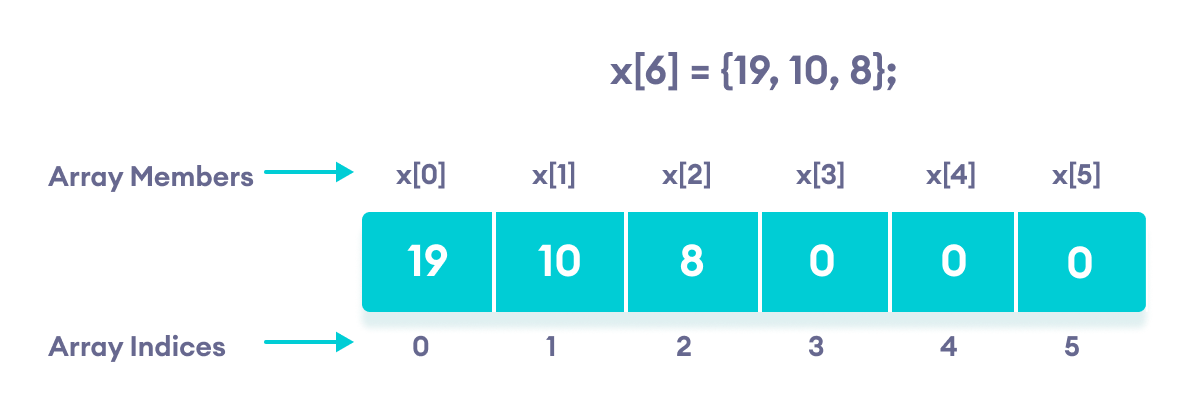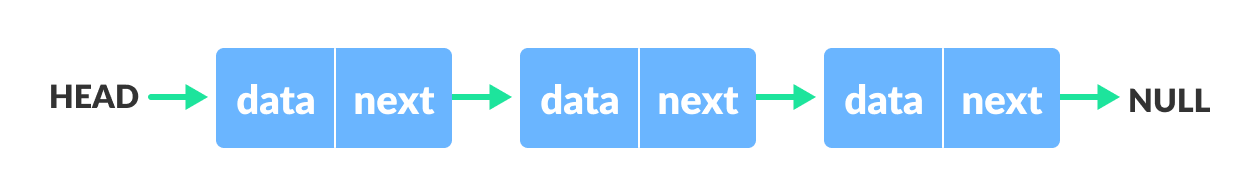# Data Structures

## Let's learn about basic data structures## What is a Data Structure?

Data Structure is nothing but storing the day in an organized way. It is used to store the data in a particular way ( such as the last input value will get out first ).

We have different data structures for different types of applications and we can use them as per our needs.

## Types of Data Structures

We have two types of data structures, they are:

1. Linear Data Structure

2. Non-Linear Data Structure

let's go with Linear Data Structure first.

## 1. Linear Data Structure:

• In a Linear Data Structure, data is stored in an ordered manner.

• We have 4 types of Linear Data Structures, they are:

1. Arrays

2. Stack

3. Queue

### 1. Arrays:

• Arrays are linear data structures, in which data is stored in order.

• Here, each data block has some address and also an indexing number.

• In arrays, data is stored in consecutive memory locations in the memory.

• For example, we define an array of type integer, and of size 5. The array name will contain the address of the first block of the array.

• We can access array elements via index numbers.### 2. Stack:

• Stack is a linear data structure, which follows the first in last out process.

• In a stack, all the elements are stored in order.

• We have one end called top in the stack where we insert and delete elements.

• We have 3 operations in Stack:

1. Push

2. Pop

3. Display

• Below is an example of a Stack:### 3. Queue:

• Queue is a linear data structure, which follows the first in first out process.

• Queue stores the data in order.

• We have 2 ends in the queue data structures, front and rear.

• At the rear end, we insert elements and at the front end we deletes an element.

• We have 3 operations in Queue:

1. Enqueue

2. Dequeue

3. Display

• Below is an example of Queue data structure:• A linked list is a linear data structure, in which we have n number of nodes and all are connected.

• In each node we have 2 cells, one cell contains the value and the second cell contains the next node address.

• The second cell of the last node contains Null, which means end of the list.

• Below is an example of a Linked list data structure:## 2. Non-Linear Data Structure:

• In a Non-Linear Data Structure, data is not stored in an ordered manner.

• We have two types of non-linear data structures:

1. Trees

2. Graphs

### 1. Trees:

• The tree is a non-linear data structure and hierarchy consisting of a collection of nodes such that each node contains a value and a list of references to other nodes.

• In trees, we have levels like the top-most parent node will be of zero level and as we go through child nodes the levels will get increase.

• Below is an example of tree data structure:### 2. Graph:

• A graph is a non-linear data structure that contains vertices and edges.

• The vertices are referred to as nodes and the edges are lines or connections between the nodes.

• Below is an example of a graph:## Outro:

• That's all about data structures for today.

• Keep Learning in Public

• Thank you...